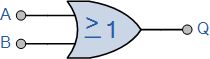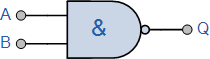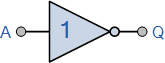# Understanding Logic Gates

Logic gates are the fundamental building blocks for digital logic or digital circuit systems. It may have multiple inputs but only single output. Output of any logic gate depends upon the logic gates expressions/symbols/logics used in that circuit. Below are the simple explanation how electronic logic gates work.

#### OR Gate

In OR gate, there are two inputs and one input. If one of the inputs is 1, the output is 1. If neither of the inputs is 1, the output is 0.

Symbol A B A+B0 0 0
0 1 1
0 1 1
1 1 1

#### AND Gate

In AND gate, there are two inputs and one input. If both inputs are 1, the output is 1. For all other cases, the output is 0.

Symbol A B AB0 0 0
0 1 0
1 0 0
1 1 1

#### NAND Gate

The NAND gate is a portmanteau of NOT and AND. It is the opposite of AND gate. It two inputs are 1, the output is 0. For other cases, the output is 0.

Symbol A B !(A.B)0 0 1
0 1 1
1 0 1
1 1 0

#### NOR Gate

The NOR gate is a portmanteau of NOT and OR. It is the opposite of OR gate. It two inputs are 1, the output is 0. For other cases, the output is 0.

Symbol A B !(A+B)0 0 1
0 1 0
1 0 0
1 1 0

#### NOT Gate

In NOT gate, there is one input and one input. The output is opposite of the input. Here are the possibilites of NOT gate in a tabular format

Symbol A !A0 1
1 0

#### XOR Gate

In XOR, the output is 0 when both inputs are 1. For all other cases, XOR gives the same output as OR.

Symbol A B !(A+B)0 0 0
1 0 1
0 1 1
1 1 0